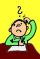# Without 2

Without multipying, tell whether the product 0.644 x 0.25 will be greater than 1 or less than 1? Explane how you know. Then find the product.

Result

p =  0.161

#### Solution:Leave us a comment of example and its solution (i.e. if it is still somewhat unclear...):

Showing 0 comments:Be the first to comment!## Next similar examples:

1. Passenger boatTwo-fifths of the passengers in the passenger boat were boys. 1/3 of them were girls and the rest were adult. If there were 60 passengers in the boat, how many more boys than adult were there?
2. CompareCompare fractions ?. Which fraction of the lower?
3. Comparing and sortingArrange in descending order this fractions: 2/7, 7/10 & 1/2
4. Milo liftedMilo lifted 65 lb 6 times and Jeremy lifted 50 lb 9 times. Which one lifted the most weight?
5. Equivalent fractionsAre these two fractions equivalent -4/9 and 11/15?
6. Equation 11Solve equation: ?
7. Double 5Peter was thinking of a number. Peter doubles it and gets an answer of 8.6. What was the original number?
8. SickSick Marcel already taken six tablets, which was a quarter of the total number of pills in the pack. How many pills were in the pack?
9. Compare:What is bigger? ?
10. To improper fractionChange mixed number to improper fraction a) 1 2/15 b) -2 15/17
11. A numberA number increased by 7.9 is 8.3
12. MidpointsTriangle whose sides are midpoints of sides of triangle ABC has a perimeter 45. How long is perimeter of triangle ABC?
13. FractionDetermine for what x fraction ?:
14. One-third 2One-third of the people in a barangay petitioned the council to allow them to plant in vacant lots and another 1/5 of the people petitioned to have a regular garbage collection. What FRACTION of the barangay population made the petition?
15. RatioIncrease in the ratio 20:4 number 18.5.
16. Dropped sheetsThree consecutive sheets dropped from the book. The sum of the numbers on the pages of the dropped sheets is 273. What number has the last page of the dropped sheets?
17. Fractions 3Calculate 1/9 of 27: